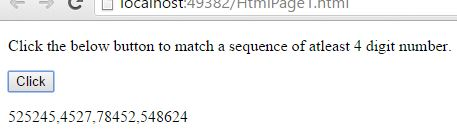# Matching atleast N digits in JavaScript

##### How to match any string that contains a sequence of atleast N digits in a string in JavaScript?

By using `d{N,}` quantifier we can match any string that contains a sequense of at least N digits in a string.

NOTE: Atleast means exactly or more, eg: atleast 5 means 5 or more.

• \d is the Metacharacter, which is used to find a digit.
• {N,} is the  input value and it must be a number.
```<p>Click the below button to match a sequence of atleast 4 digit number.</p>
<input type="button" value="Click" onclick="mySearch()" />
<p id="myId"></p>

<script>
function mySearch() {
var a = "525245, 4527, 651, 78452, 35, 4, 548624";
var b = /\d{4,}/g;
var r = a.match(b);
document.getElementById("myId").innerHTML = r;
}
</script>
```

In the above code snippet we have given `Id` as "`myId`"to the second `<p>` element in the HTML code. There is a function mySearch() in the`<script>`block which is connected to the onclick of the HTML button and there is a string with value "525245, 4527, 651, 78452, 32, 4, 548624" to the variable `a`we need to match any string that contains a sequence of atleast N digits, for that we are using `d{N,}` quantifier in variable `b``var b = /\d{4,}/g` here `\d` is the metacharacter for finding a digit, {4,} is the input value of `{N,}` for matching any string that contains a sequence of atleast 4-digit numbers and `g` do the global search in a string, varaible `r` returns the matched sequence result. Onclick of the button "Click" in the HTML code fires the function mySearch() in the `<script>` block at the same time `d{N,}` quantifier matches any string that contains a sequence of atleast  N digits (N=4 & N,=4 or more) in a string with the help of global search `g` and gives the output.

OUTPUTViews: 3192 | Post Order: 148